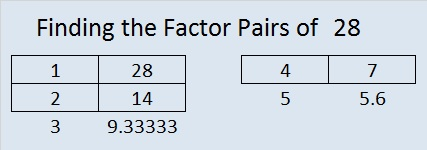# 28 and Level 5 Snowflake

28 is a composite number. 28 = 1 x 28, 2 x 14, or 4 x 7. Factors of 28: 1, 2, 4, 7, 14, 28. Prime factorization: 28 = 2 x 2 x 7, which can also be written 28 = 2² x 7.When 28 is a clue in the FIND THE FACTORS puzzles, the factors will be 4 and 7.This snowflake is actually a logic puzzle based on the multiplication table. Because it is a level 5 puzzle, it can be a little tricky to solve, and isn’t for beginners. If you haven’t solved a Find the Factors puzzle before, this level 2 snowflake puzzle might be a better choice:How do you solve a level 5 FIND THE FACTORS 1 – 12 puzzle? As always the object is to write the numbers 1-12 in the top row and again in the first column so that those numbers are the factors of the given clues. Use logic, not guessing and checking, to find its unique solution. Here is how to solve a level 5 puzzle:

Begin by looking for perfect square clues, 1, 25, 49, 64, 81, 100, 121, or 144, because there is only one way to factor any of them so that both factors are numbers from 1 to 12. IF you find one of THOSE perfect square clues, write down its factors in the corresponding factor row and factor column.

Next locate a row or a column with at least 2 clues. Find ALL the common factors of that row or column that will allow you to write only numbers from 1 to 12 in both the factor row and the factor column. If that row or column has more than one common factor, leave it alone for now. In a level 5 puzzle there will be at least one row or column that has only one common factor. When you find such a row or column, write its factors in the corresponding factor cells.

Let’s use the level 5 puzzle from wk 1 – 2014 as an example. Starting at the top of the puzzle look at all each row to see if it has more than one clue. We notice that there are two rows with 2 clues, but in both cases, there is more than one possible common factor, so we will ignore those rows for now. Starting on the left, look at each column to see if it has more than one clue. There are 2 columns that have 2 clues, and one of those columns has 3 possible common factors. If you were to guess which of those factors were correct, you would have a 67% chance of guessing wrong. Guessing and checking leads to frustration. Use logic and start with the column that has only one possible common factor, in this case: 1.Now you have at least 3 factors written down. Next look at ALL the remaining clues in the puzzle and find one that can only be factored using one of the factors you’ve written down already but haven’t used twice. (You notice that 54 = 9 x 6, so you can write 9 and 6 in the appropriate cells.)

Sometime during this process, you will discover that one or more of the remaining rows or columns that had more than one clue have had some of the possible factors eliminated. Logic, or the process of elimination, will help you know the only common factor that will work for those clues in this puzzle. (In last week’s puzzle, you may notice that 28 = 4 x 7. That means that 16 cannot be 4 x 4 because we can’t have any factor used more than one time in either the factor row or the factor column. That means in this particular puzzle 16 = 8 x 2. Since you already found one 8, it is easy to place this 8 and the 2.)

Continue to look at all the clues and use logic until all (or almost all) of the factors are found.

If a row or a column contains no clues, use logic is determine which number from 1 to 12 (or 1 to 10) should be written there.

While working on these puzzles, occasionally count from 1 to 12 (1 to 10) as you examine numbers in the factor row and factor column to make sure that a number is written only once in either place. Good luck!

This week’s puzzles are also available in an excel file here, if you have a spreadsheet program on your computer you can access it. If you enable editing in excel, you can type your answers directly onto the puzzle, and you can also easily print the puzzles. Here is the solution to the Week 1 – 2014, Level 5 puzzle:This site uses Akismet to reduce spam. Learn how your comment data is processed.